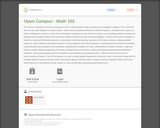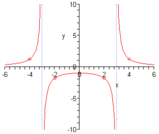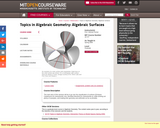Updating search results...

# 3 Results

View
Selected filters:
• rationalOnly Sharing Permitted
CC BY-NC-ND
Rating
0.0 stars

This course is designed to take the concepts you learn in developmental math to expand your knowledge of algebra. This course will focus on two major algebraic concepts to learn - how to SOLVE equations and how to GRAPH equations. Throughout this course you will be challenged to recall ALL of your prior knowledge of operations of real numbers as well as your knowledge related to solving and graphing linear equations (which you should have already mastered from developmental algebra). You will use this prior knowledge to expand on learning the following objectives: solving linear & rational equations. operations of complex numbers, solving quadratic equations, solving radical & polynomial equations, solving equations with rational exponents, solving linear and compound inequalities, solving absolute value equations and inequalities, graphing linear equations & slope, understanding concepts of domain, range and function notation, finding compositions of functions, finding inverses of functions, solving and graphing exponential and logarithmic equations, solving and graphing systems of equations and inequalities, and graphing conics.

*Open Campus courses are non-credit tutorials and cannot, in and of themselves, be used to satisfy degree requirements at Bossier Parish Community College (BPCC). (College Algebra Course by Bossier Parish Community College is licensed under a Creative Commons Attribution-NonCommercial-NoDerivatives 4.0 International License. Based on a work at http://bpcc.edu/opencampus/index.html.)

Subject:
Mathematics
Material Type:
Full Course
Provider:
Bossier Parish Community College
Author:
Stacey Black
07/05/2018Conditional Remix & Share Permitted
CC BY-NC
Rating
0.0 stars

This is a lesson plan that helps students identify the features of rational functions before they graph the rational functions.&nbsp; They will identify the holes, horizontal and vertical asymptotes, domain and range, and the x and y intercepts.

Subject:
Secondary Mathematics
Material Type:
Lesson Plan
Author:
Tyrani Bevell
10/02/2021Conditional Remix & Share Permitted
CC BY-NC-SA
Rating
0.0 stars

The main aims of this seminar will be to go over the classification of surfaces (Enriques-Castelnuovo for characteristic zero, Bombieri-Mumford for characteristic p), while working out plenty of examples, and treating their geometry and arithmetic as far as possible.

Subject:
Mathematics
Material Type:
Full Course
Provider:
M.I.T.
Provider Set:
M.I.T. OpenCourseWare
Author:
Kumar, Abhinav